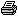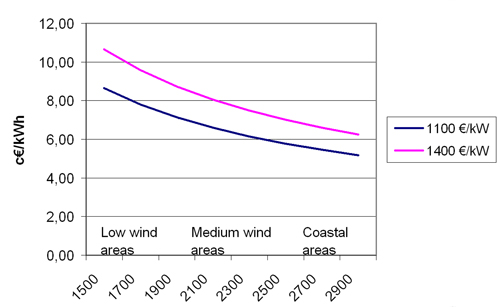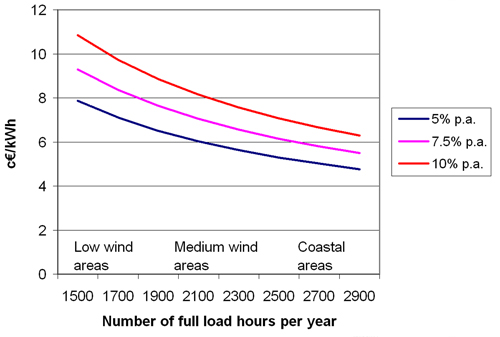# MAIN PUBLICATION :

 Home » ECONOMICS » Cost of on-land wind power » The cost of energy generated by wind power### The cost of energy generated by wind power

The total cost per kWh produced (unit cost) is calculated by discounting and levelising investment and O&M costs over the lifetime of the turbine, and then dividing them by the annual electricity production. The unit cost of generation is thus calculated as an average cost over the turbine’s lifetime. In reality, actual costs will be lower than the calculated average at the beginning of the turbine’s life, due to low O&M costs, and will increase over the period of turbine use.

The turbine’s power production is the single most important factor for the cost per unit of power generated. The profitability of a turbine depends largely on whether it is sited at a good wind location. In this section, the cost of energy produced by wind power will be calculated according to a number of basic assumptions. Due to the importance of the turbine’s power production, the sensitivity analysis will be applied to this parameter. Other assumptions include the following:

• Calculations relate to new land-based, medium-sized turbines (1.5-2 MW) that could be erected today.
• Investment costs reflect the range given in Section 2 - that is, a cost per kW of 1,100-1,400 €/kW, with an average of 1,225 €/kW. These costs are based on data from IEA and stated in 2006 prices.
• Operation and maintenance costs are assumed to be 1.45 c€/kWh as an average over the lifetime of the turbine.
• The lifetime of the turbine is set at 20 years, in accordance with most technical design criteria.
• The discount rate is assumed to range from 5-10% per annum. In the basic calculations, a discount rate of 7.5% per annum is used, although a sensitivity analysis of the importance of this interest range is also performed.
• Economic analyses are carried out on a simple national economic basis. Taxes, depreciation and risk premiums are not taken into account and all calculations are based on fixed 2006 prices.

The calculated costs per kWh of wind-generated power, as a function of the wind regime at the chosen sites are shown in Figure 1.8 below. As illustrated, the costs range from approximately 7-10 c€/kWh at sites with low average wind speeds, to approximately 5-6.5 c€/kWh at windy coastal sites, with an average of approximately 7c€/kWh at a wind site with average wind speeds.

In Europe, the good coastal positions are located mainly on the coasts of the UK, Ireland, France, Denmark and Norway. Medium wind areas are mostly found inland in mid and southern Europe - Germany, France, Spain, Holland and Italy; and also in Northern Europe in Sweden, Finland and Denmark. In many cases, local conditions significantly influence the average wind speeds at a specific site, so significant fluctuations in the wind regime are to be expected even for neighbouring areas.Figure 1.8: Calculated costs per kWh of wind generated power as a function of the wind regime at the chosen site (number of full load hours).Source Risoe. Assumptions: see text above. Note: In this figure, the number of full load hours is used to represent the wind regime. Full load hours are calculated as the turbine’s average annual production divided by its rated power. The higher the number of full load hours, the higher the wind turbine’s production at the chosen site.

Approximately 75-80% of total power production costs for a wind turbine are related to capital costs - that is, the costs of the turbine, foundation, electrical equipment and grid connection. Thus, a wind turbine is capital intensive compared with conventional fossil fuel-fired technologies, such as natural gas power plants, where as much as 40-60% of total costs are related to fuel and operation and maintenance costs. For this reason, the costs of capital (discount or interest rate) are an important factor for the cost of wind generated power; a factor which varies considerably between the EU member countries.

In Figure 1.9, the costs per kWh of wind-produced power are shown as a function of the wind regime and the discount rate (which varies between 5 and 10% per annum).Figure 1.9: The costs of wind produced power as a function of wind speed (number of full load hours) and discount rate. The installed cost of wind turbines is assumed to be 1,225 €/kW. Source: Risoe

As illustrated, the costs ranges between around 6 and 8 c€/kWh at medium wind positions, indicating that a doubling of the interest rate induces an increase in production costs of 2 c€/kWh. In low wind areas, the costs are significantly higher, at around 8-11 c€/kWh, while the production costs range between 5 and 7 c€/kWh in coastal areas.

 Sitemap | Partners | Disclaimer | Contact coordinated bysupported byThe sole responsibility for the content of this webpage lies with the authors. It does not necessarily reflect the opinion of the European Communities. The European Commission is not responsible for any use that maybe made of the information contained therein.